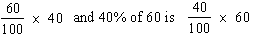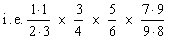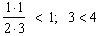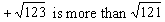Please Support the Monkey! Tell All your Friends and Teachers
 ANSWER EXPLANATIONS Test Paper I : Section II : Quantitative Section 60% of 40% isboth of which are obviously equal and this can be concluded without doing any calculations. Hence C. The numbers given in column A can be rearranged as follows:each of these is a proper fractionand so on i.e. in each case the numerator is less than the denominator and hence each fraction is less than the product of such fractions will also be less than one. Hence B.i.e. more than 11. Hence A. Test 1 Section 1 : Verbal Section Section 2 : Quantitative Section Section 3 : Analytical Section Section 4 : Quantitative Section Section 5 : Verbal Section Section 6 : Analytical Section Section 7 : Verbal Section Answer Key To Test 1 Answer Explanation To Test 1 Section 1 : Verbal Section Section 2 : Quantitative Section Section 3 : Analytical Section Section 4 : Quantitative Section Section 5 : Verbal Section Section 6 : Analytical Section Section 7 : Verbal Section